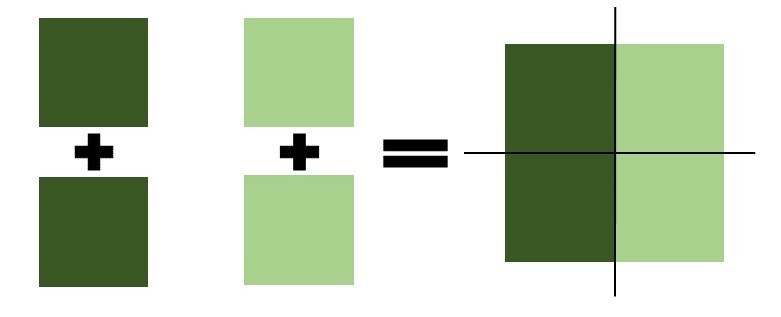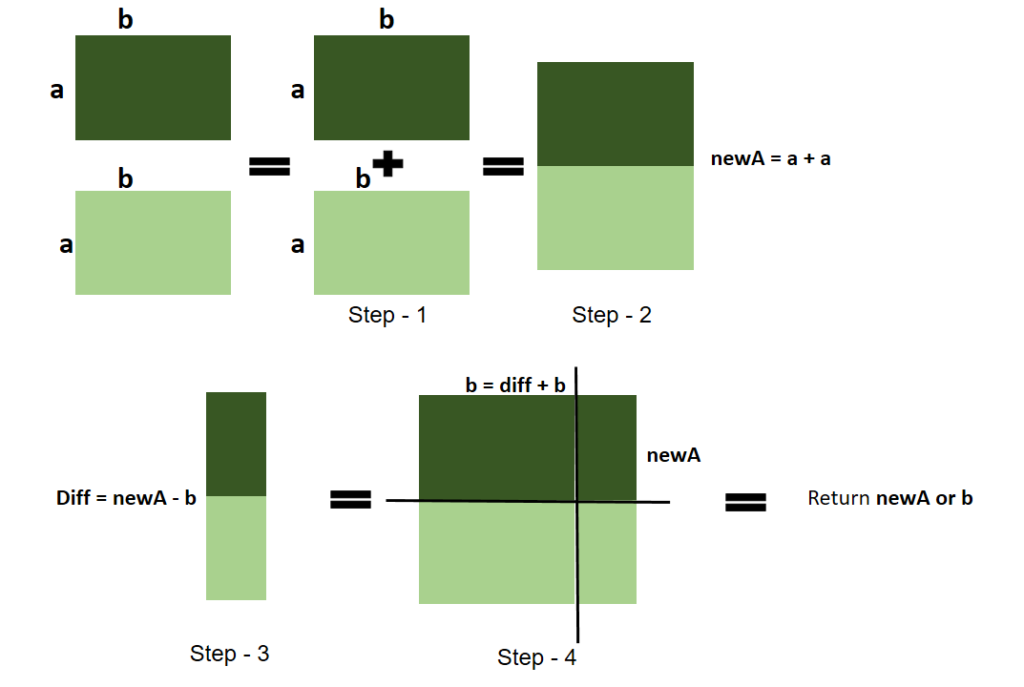Related Articles

# Find side of Square which makes minimal area to fit two identical rectangles inside it

• Last Updated : 07 Apr, 2021

Given height H, and width W, of a rectangle, the task is to find the side of a square of the minimum area in which two rectangles fit completely.

Note:

1. Two rectangles can touch each other by side or corner.
2. Rectangles cannot intersect each other.
3. Rectangles can also touch the sides of the square but must be completely inside it.
4. The Rectangles can be rotate also

Example :

Input: H = 4, W = 2
Output:
Explanation:
Minimum side of the square is 4
Input: H = 4, W = 4
Output: 8

Approach :
There are three possible cases:

1. At first check the given height and width is same or not, if same then rectangles are itself a square, so simply attach two squares and double the side,1. Then if 1st case not satisfied then check if height > width or not,
if greater, then double the width value and add it to the width and make a new width,

new_Width = width + width

• Then find the difference of ‘new_Width’ and ‘height’ and store the difference in a new variable “diff”,
• If the ‘new_Width’ is less than ‘height’, then add the “diff” value to the ‘new_Width’, then the ‘height’ and ‘new_width’ are same and return ‘new_Width’, that is the side of the minimal square.
• If the ‘new_Width’ is greater than ‘height’, then add the “diff” value to the ‘height’, then the ‘height’ and ‘width’ are same, and return ‘height’, that is the side of the minimal square.

1. Then if 2nd case not satisfied then check if height < width
if smaller, then double the ‘height’ value and add it to the height and make a new height,

new_Height = height + height

• Then find the difference of ‘new_Height’ and ‘width’ and store the difference in a new variable “diff”,
• If the ‘new_Height’ is less than ‘width’, then add the “diff” value to the ‘new_Height’, then the ‘width’ and ‘new_Height’ are same and return ‘new_Height’, that is the side of the minimal square.
• If the ‘new_Height’ is greater than ‘width’, then add the “diff” value to the ‘width’, then the ‘height’ and ‘width’ are same, and return ‘height’, that is the side of the minimal square.

1. EndBelow is the implementation of the above approach:

## C++

 `// C++ program of the above approach` `#include ``using` `namespace` `std;` `int` `minimalSquareSide(``int` `a, ``int` `b)``{``    ``// if 'a' and 'b' same``    ``// then double 'a' or 'b'``    ``// and return (2*a) or (2*b)``    ``if` `(a == b) {``        ``return` `2 * a;``    ``}` `    ``// check if a!=b``    ``if` `(a != b) {``        ``// if a > b``        ``if` `(a > b) {` `            ``// double the smaller value``            ``// that is 'b' and store``            ``// it to 'newB'``            ``int` `newB = b + b;` `            ``// find the difference of``            ``// 'newB and 'a'``            ``int` `diff = ``abs``(newB - a);` `            ``// if 'newB' < a``            ``if` `(newB < a) {``                ``// then add the difference of``                ``// 'newB' and 'a' to the 'b'``                ``// to make 'b' and 'a' as same``                ``b = newB + diff;` `                ``// return side of the``                ``// square a or b``                ``if` `(a == b)``                    ``return` `a;``                ``return` `0;``            ``}` `            ``else` `{``                ``// if 'newB' > a then``                ``// then add the difference``                ``// of 'newB' and 'a' to``                ``// the 'a' to make 'a' and``                ``// 'newB' as same``                ``a = a + diff;` `                ``// return side of the``                ``// square a or newB``                ``if` `(a == newB)``                    ``return` `a;``                ``return` `0;``            ``}``        ``}` `        ``// if a < b``        ``else` `{``            ``// double the smaller value``            ``// that is 'a' and store``            ``// it to 'newA'``            ``int` `newA = a + a;` `            ``// find the difference of``            ``// 'newA and 'b'``            ``int` `diff = ``abs``(newA - b);` `            ``// if 'newA' < b``            ``if` `(newA < b) {``                ``// then add the difference``                ``// of 'newA' and 'b' to``                ``// the 'a' to make 'a'``                ``// and 'b' as same``                ``a = diff + newA;` `                ``// return side of the``                ``// square a or b``                ``if` `(a == b)``                    ``return` `a;``                ``return` `0;``            ``}` `            ``else` `{``                ``// if 'newA' > b then``                ``// then add the difference``                ``// of 'newA' and 'b' to``                ``// the 'b' to make 'b' and``                ``// 'newA' as same``                ``b = b + diff;` `                ``// return side of the``                ``// square b or newA``                ``if` `(b == newA)``                    ``return` `b;``                ``return` `0;``            ``}``        ``}``    ``}``}` `// Drive Code``int` `main()``{``    ``int` `H, W;` `    ``// Size of rectangle``    ``H = 3, W = 1;` `    ``cout << minimalSquareSide(H, W) << endl;``    ``return` `0;``}`

## Java

 `// Java program of the above approach``class` `GFG{``    ` `public` `static` `int` `minimalSquareSide(``int` `a, ``int` `b)``{``    ` `    ``// If 'a' and 'b' same``    ``// then double 'a' or 'b'``    ``// and return (2*a) or (2*b)``    ``if` `(a == b)``    ``{``        ``return` `2` `* a;``    ``}``    ` `    ``// Check if a!=b``    ``if` `(a != b)``    ``{``        ` `        ``// If a > b``        ``if` `(a > b)``        ``{``            ` `            ``// Double the smaller value``            ``// that is 'b' and store``            ``// it to 'newB'``            ``int` `newB = b + b;``    ` `            ``// Find the difference of``            ``// 'newB and 'a'``            ``int` `diff = Math.abs(newB - a);``    ` `            ``// If 'newB' < a``            ``if` `(newB < a)``            ``{``                ` `                ``// Then add the difference of``                ``// 'newB' and 'a' to the 'b'``                ``// to make 'b' and 'a' as same``                ``b = newB + diff;``    ` `                ``// Return side of the``                ``// square a or b``                ``if` `(a == b)``                    ``return` `a;``                ``return` `0``;``            ``}``            ``else``            ``{``                ` `                ``// If 'newB' > a then``                ``// then add the difference``                ``// of 'newB' and 'a' to``                ``// the 'a' to make 'a' and``                ``// 'newB' as same``                ``a = a + diff;``    ` `                ``// Return side of the``                ``// square a or newB``                ``if` `(a == newB)``                    ``return` `a;``                ``return` `0``;``            ``}``        ``}``    ` `        ``// If a < b``        ``else``        ``{``            ` `            ``// Double the smaller value``            ``// that is 'a' and store``            ``// it to 'newA'``            ``int` `newA = a + a;``    ` `            ``// Find the difference of``            ``// 'newA and 'b'``            ``int` `diff = Math.abs(newA - b);``    ` `            ``// If 'newA' < b``            ``if` `(newA < b)``            ``{``                ` `                ``// Then add the difference``                ``// of 'newA' and 'b' to``                ``// the 'a' to make 'a'``                ``// and 'b' as same``                ``a = diff + newA;``    ` `                ``// Return side of the``                ``// square a or b``                ``if` `(a == b)``                    ``return` `a;``                ``return` `0``;``            ``}``            ``else``            ``{``                ` `                ``// If 'newA' > b then``                ``// then add the difference``                ``// of 'newA' and 'b' to``                ``// the 'b' to make 'b' and``                ``// 'newA' as same``                ``b = b + diff;``    ` `                ``// Return side of the``                ``// square b or newA``                ``if` `(b == newA)``                    ``return` `b;``                ``return` `0``;``            ``}``        ``}``    ``}``    ``return` `0``;``}` `// Driver code   ``public` `static` `void` `main(String[] args)``{``    ``int` `H, W;` `    ``// Size of rectangle``    ``H = ``3``; W = ``1``;``    ` `    ``System.out.println(minimalSquareSide(H, W));``}``}` `// This code is contributed by divyeshrabadiya07`

## Python3

 `# Python3 program for the above approach` `def` `minimalSquareSide(a, b):``    ` `    ``# If 'a' and 'b' same``    ``# then double 'a' or 'b'``    ``# and return (2*a) or (2*b)``    ``if` `(a ``=``=` `b):``        ``return` `2` `*` `a` `    ``# Check if a!=b``    ``if` `(a !``=` `b):``        ` `        ``# If a > b``        ``if` `(a > b):` `            ``# Double the smaller value``            ``# that is 'b' and store``            ``# it to 'newB'``            ``newB ``=` `b ``+` `b` `            ``# Find the difference of``            ``# 'newB and 'a'``            ``diff ``=` `abs``(newB ``-` `a)` `            ``# If 'newB' < a``            ``if` `(newB < a):``                ` `                ``# Then add the difference of``                ``# 'newB' and 'a' to the 'b'``                ``# to make 'b' and 'a' as same``                ``b ``=` `newB ``+` `diff` `                ``# Return side of the``                ``# square a or b``                ``if` `(a ``=``=` `b):``                    ``return` `a``                ``return` `0` `            ``else``:``                ` `                ``# If 'newB' > a then``                ``# then add the difference``                ``# of 'newB' and 'a' to``                ``# the 'a' to make 'a' and``                ``# 'newB' as same``                ``a ``=` `a ``+` `diff` `                ``# Return side of the``                ``# square a or newB``                ``if` `(a ``=``=` `newB):``                    ``return` `a``                ``return` `0` `        ``# If a < b``        ``else``:``            ` `            ``# Double the smaller value``            ``# that is 'a' and store``            ``# it to 'newA'``            ``newA ``=` `a ``+` `a` `            ``# Find the difference of``            ``# 'newA and 'b'``            ``diff ``=` `abs``(newA ``-` `b)` `            ``# If 'newA' < b``            ``if` `(newA < b):``                ` `                ``# Then add the difference``                ``# of 'newA' and 'b' to``                ``# the 'a' to make 'a'``                ``# and 'b' as same``                ``a ``=` `diff ``+` `newA` `                ``# Return side of the``                ``# square a or b``                ``if` `(a ``=``=` `b):``                    ``return` `a``                ``return` `0``            ` `            ``else``:``                ` `                ``# If 'newA' > b then``                ``# then add the difference``                ``# of 'newA' and 'b' to``                ``# the 'b' to make 'b' and``                ``# 'newA' as same``                ``b ``=` `b ``+` `diff` `                ``# Return side of the``                ``# square b or newA``                ``if` `(b ``=``=` `newA):``                    ``return` `b``                ``return` `0` `# Driver code` `# Size of rectangle``H ``=` `3``W ``=` `1` `print``(minimalSquareSide(H, W))` `# This code is contributed by sanjoy_62`

## C#

 `// C# program of the above approach``using` `System;` `class` `GFG{``    ` `public` `static` `int` `minimalSquareSide(``int` `a, ``int` `b)``{``    ` `    ``// If 'a' and 'b' same``    ``// then double 'a' or 'b'``    ``// and return (2*a) or (2*b)``    ``if` `(a == b)``    ``{``        ``return` `2 * a;``    ``}``    ` `    ``// Check if a!=b``    ``if` `(a != b)``    ``{``        ` `        ``// If a > b``        ``if` `(a > b)``        ``{``            ` `            ``// Double the smaller value``            ``// that is 'b' and store``            ``// it to 'newB'``            ``int` `newB = b + b;``    ` `            ``// Find the difference of``            ``// 'newB and 'a'``            ``int` `diff = Math.Abs(newB - a);``    ` `            ``// If 'newB' < a``            ``if` `(newB < a)``            ``{``                ` `                ``// Then add the difference of``                ``// 'newB' and 'a' to the 'b'``                ``// to make 'b' and 'a' as same``                ``b = newB + diff;``    ` `                ``// Return side of the``                ``// square a or b``                ``if` `(a == b)``                    ``return` `a;``                ``return` `0;``            ``}``            ``else``            ``{``                ` `                ``// If 'newB' > a then``                ``// then add the difference``                ``// of 'newB' and 'a' to``                ``// the 'a' to make 'a' and``                ``// 'newB' as same``                ``a = a + diff;``    ` `                ``// Return side of the``                ``// square a or newB``                ``if` `(a == newB)``                    ``return` `a;``                ``return` `0;``            ``}``        ``}``    ` `        ``// If a < b``        ``else``        ``{``            ` `            ``// Double the smaller value``            ``// that is 'a' and store``            ``// it to 'newA'``            ``int` `newA = a + a;``    ` `            ``// Find the difference of``            ``// 'newA and 'b'``            ``int` `diff = Math.Abs(newA - b);``    ` `            ``// If 'newA' < b``            ``if` `(newA < b)``            ``{``                ` `                ``// Then add the difference``                ``// of 'newA' and 'b' to``                ``// the 'a' to make 'a'``                ``// and 'b' as same``                ``a = diff + newA;``    ` `                ``// Return side of the``                ``// square a or b``                ``if` `(a == b)``                    ``return` `a;``                ``return` `0;``            ``}``            ``else``            ``{``                ` `                ``// If 'newA' > b then``                ``// then add the difference``                ``// of 'newA' and 'b' to``                ``// the 'b' to make 'b' and``                ``// 'newA' as same``                ``b = b + diff;``    ` `                ``// Return side of the``                ``// square b or newA``                ``if` `(b == newA)``                    ``return` `b;``                ``return` `0;``            ``}``        ``}``    ``}``    ``return` `0;``}` `// Driver code``public` `static` `void` `Main(String[] args)``{``    ``int` `H, W;` `    ``// Size of rectangle``    ``H = 3; W = 1;``    ` `    ``Console.WriteLine(minimalSquareSide(H, W));``}``}` `// This code is contributed by sapnasingh4991`

## Javascript

 ``
Output:
`3`

Time complexity: O(1)
Auxiliary Space: O(1)My Personal Notes arrow_drop_up